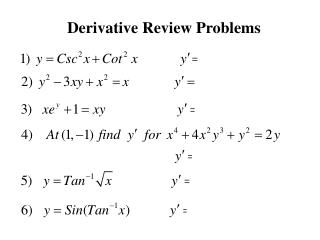DownloadDownload PresentationDerivative Review Problems

# Derivative Review Problems

Download Presentation## Derivative Review Problems

- - - - - - - - - - - - - - - - - - - - - - - - - - - E N D - - - - - - - - - - - - - - - - - - - - - - - - - - -
##### Presentation Transcript

1. Derivative Review Problems

2. Wouldn’t it be great if everybody in the world lightened up? Ignore the occasional low Calculus grade Why can’t we Get along? Live and Let live Always wait for the context Let bygones Be bygones

3. Calculus 2413 Chapter 3(10) Related Rates

4. When a pool is filled the change in the depth is related to the change in the volume. You might consider When a ladder leans against a building and the bottom (x) is moved away from the building the top (y) will move down. You might consider Related Rates Used when you have change related to time

5. Take a time derivative of each:

6. Related Rates - Steps • Draw a picture and find or write an equation relating two variables. 2) Take a time derivative of the equation. 3) Put in values that you know and solve for what you are missing.

7. Example 1: Find the rate of change in the distance between the origin and a moving point on the graph of:

8. Example 2: The diameter of a circle is increasing at a rate of 4 ft/sec. How fast is the area increasing when the diameter is 10?

9. Example 3: Water is being poured into an inverted cone at a rate of 10 ft3/sec. At the top, the radius of the cone is 8 and the depth is 20 feet. How fast is the depth rising when the water is 7 feet deep?

10. A 30 ft. ladder is leaning against a building and the bottom is pulled out at a rate of 3 ft/min. How fast is the top of the ladder moving down when the bottom is 12 ft. from the building? Example 4: 27.5 12